# Spatial Uncertainty Propagation Analysis

### Case study with spatially variable standard deviation - slope calculations with a digital elevation model (DEM)

#### 2020-04-29

Sys.sleep(100)

# Case study with spatially variable standard deviation - slope calculations

### Introduction/Problem definition

In many geographical studies a DEM is a critical variable, because DEM-derived variables, such as slope, aspect, curvature and viewshed are of great importance in many types of analysis. However, a DEM is only an approximation of the real elevation in the area. It contains errors. Insight into DEM error (uncertainty) propagation through the calculation of, for example, slope, is therefore crucial. We can use the Monte Carlo (MC) method to analyse how the error propagates through spatial operations and models. This method is briefly described below.

The MC method is fairly straightforward in application, but in case of spatially distributed variables like elevation one should consider taking spatial autocorrelation into account. That is because the model output uncertainty may be influenced by the spatial correlation in the input. For example, slope calculations are quite sensitive to the degree of spatial autocorrelation in DEM uncertainty (Heuvelink, 1998).

### Monte Carlo methodology for spatial uncertainty analysis with spatially variable sd

The uncertainty propagation analysis approach applied here is based on the Monte Carlo method that computes the output of the model repeatedly, with input values that are randomly sampled from their marginal or joint probability distribution function (pdf). The set of model outputs forms a random sample from the output pdf, so that parameters of the distribution, such as the mean, variance and quantiles, can be estimated from the sample. The method thus consists of the following steps:

1. Characterise uncertain model inputs with (spatial) pdfs.
2. Repeatedly sample from (spatial) pdfs of uncertain inputs.
3. Run model with sampled inputs and store model outputs.
4. Compute summary statistics of model outputs.

Note that the above ignores uncertainty in model parameters and model structure, but these can easily be included if available as pdfs. A random sample from the model inputs can be obtained using an appropriate pseudo-random number generator.

For uncertain spatially distributed continuous variables, such as elevation, we assume the following geostatistical model:

Z(x)= μ(x)+ σ(x)∙ε(x)

where x is geographic location, μ is the (deterministic) mean of Z, σ is its standard deviation and ε is a standard normal (hence zero mean and unit variance), second-order stationary stochastic residual, whose spatial autocorrelation is modelled with a semivariogram or correlogram. Both μ and σ may vary in space so that spatial trends and spatially variable uncertainty can be taken into account. In the case of elevation, it makes sense to let μ be equal to the DEM while σ may have greater values in mountainous areas than in flat terrain (e.g. Beekhuizen, et al., 2011). In the example below both maps have been prepared. The random sample is drawn from the pdf of ε to further calculate a sample from Z.

### DEM uncertainty propagation analysis with ‘spup’

#### Preliminaries - load and view the data

The example data for slope calculations are a 30m resolution mean DEM and standard deviation from the Zlatibor region in Serbia (Hengl et al., 2008).

The data contain two spatial objects: a mean (dem30m) and the standard deviation (dem30m_sd) map. A function that calculates slope from elevation that will be used later is also provided.

# load packages
library(sp)
library(spup)
library(GGally)
library(gridExtra)
library(purrr)
library(magrittr)

# source code for Slope model
source("examples/Slope.R")

# set seed
set.seed(12345)

# load and view the data
data(dem30m, dem30m_sd)
str(dem30m)
Formal class 'SpatialGridDataFrame' [package "sp"] with 4 slots
[email protected] data       :'data.frame': 15000 obs. of  1 variable:
.. ..$Elevation: num [1:15000] 1010 1010 1008 1007 1005 ... [email protected] grid :Formal class 'GridTopology' [package "sp"] with 3 slots .. .. [email protected] cellcentre.offset: Named num [1:2] 7394264 4842014 .. .. .. ..- attr(*, "names")= chr [1:2] "x" "y" .. .. [email protected] cellsize : num [1:2] 30 30 .. .. [email protected] cells.dim : int [1:2] 150 100 [email protected] bbox : num [1:2, 1:2] 7394249 4841999 7398749 4844999 .. ..- attr(*, "dimnames")=List of 2 .. .. ..$ : chr [1:2] "x" "y"
.. .. ..$: chr [1:2] "min" "max" [email protected] proj4string:Formal class 'CRS' [package "sp"] with 1 slot .. .. [email protected] projargs: chr "+init=epsg:3857 +proj=merc +a=6378137 +b=6378137 +lat_ts=0.0 +lon_0=0.0 +x_0=0.0 +y_0=0 +k=1.0 +units=m +nadgri"| __truncated__ str(dem30m_sd) Formal class 'SpatialGridDataFrame' [package "sp"] with 4 slots [email protected] data :'data.frame': 15000 obs. of 1 variable: .. ..$ Elevation_sd: num [1:15000] 0.1 0.1 0.1 0.1 0.1 0.1 0.1 0.1 0.1 0.1 ...
[email protected] grid       :Formal class 'GridTopology' [package "sp"] with 3 slots
.. .. [email protected] cellcentre.offset: Named num [1:2] 7394264 4842014
.. .. .. ..- attr(*, "names")= chr [1:2] "x" "y"
.. .. [email protected] cellsize         : num [1:2] 30 30
.. .. [email protected] cells.dim        : int [1:2] 150 100
[email protected] bbox       : num [1:2, 1:2] 7394249 4841999 7398749 4844999
.. ..- attr(*, "dimnames")=List of 2
.. .. ..$: chr [1:2] "s1" "s2" .. .. ..$ : chr [1:2] "min" "max"
[email protected] proj4string:Formal class 'CRS' [package "sp"] with 1 slot
.. .. [email protected] projargs: chr "+init=epsg:3857 +proj=merc +a=6378137 +b=6378137 +lat_ts=0.0 +lon_0=0.0 +x_0=0.0 +y_0=0 +k=1.0 +units=m +nadgri"| __truncated__
grid.arrange(spplot(dem30m, main = list(label = "Mean DEM [m]", cex = 1)),
spplot(dem30m_sd, main = list(label = "DEM sd [m]", cex = 1)))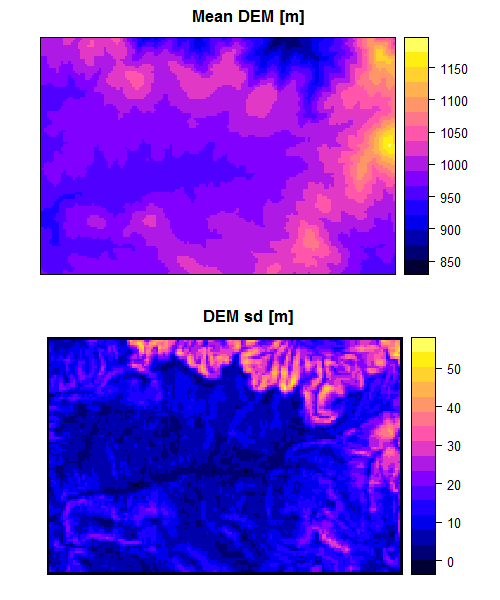#### Define uncertainty model (UM) for elevation

The first step in uncertainty propagation analysis is to define an uncertainty model for the uncertain input variable, here elevation, that will be used in the Monte Carlo uncertainty propagation analysis.

In case of elevation, the ε(x) are spatially correlated and in order to include this in the analysis, we need to describe it by spatial correlogram parameters. The makeCRM() function collates all necessary information into a list.

Let us assume that the spatial autocorrelation of the DEM errors is an exponentially decreasing function with a short-distance correlation of 0.8 and a range parameter of 300m.

# define spatial correlogram model
dem_crm <- makeCRM(acf0 = 0.8, range = 300, model = "Exp")

We can view the correlogram by plotting it.

plot(dem_crm, main = "DEM correlogram")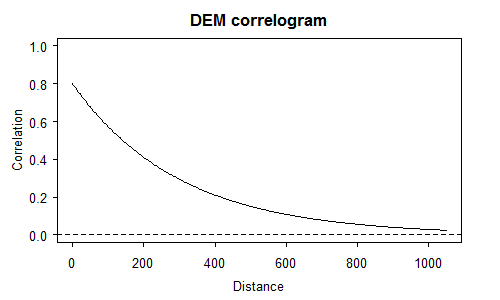Spatial correlograms summarise patterns of spatial autocorrelation in data and model residuals. They show the degree of correlation between values at two locations as a function of the separation distance between the locations. In the case above the correlation declines with distance, as is usually the case. The correlation becomes negligibly small for distances greater than 800 m. Notice also that the correlation is not perfect at distances close to zero. This signifies the so-called ‘nugget’ effect. The nugget effect, shape of the correlation function and maximum range can be modified by changing the parameters of the makeCRM() function. Try, for example, these combinations and see how they look like:

par(mfrow = c(2, 2))
crm <- makeCRM(acf0 = 0.8, range = 700, model = "Sph")
plot(crm, main = "'Spherical', acf0 = 0.8, range = 700")
crm <- makeCRM(acf0 = 0.2, range = 700, model = "Sph")
plot(crm, main = "'Spherical', acf0 = 0.2, range = 700")
crm <- makeCRM(acf0 = 0.8, range = 700, model = "Lin")
plot(crm, main = "'Linear', acf0 = 1.0, range = 700")
crm <- makeCRM(acf0 = 0.8, range = 200, model = "Gau")
plot(crm, main = "'Gaussian', acf0 = 0.8, range = 200")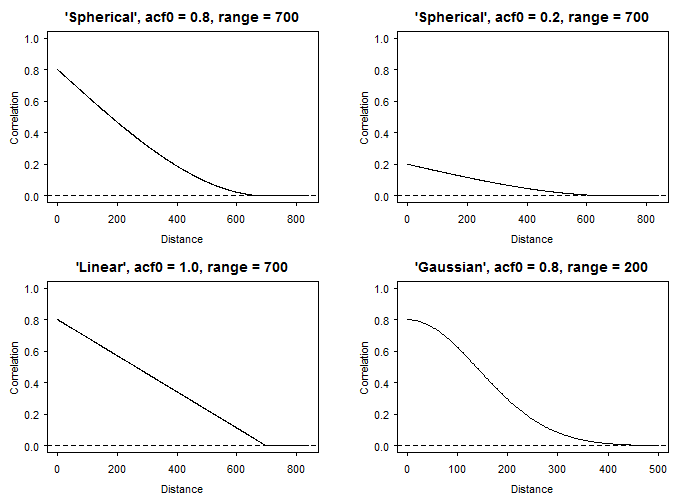In order to complete the description of the uncertain variable we use the defineUM() function that collates all information about the DEM uncertainty into one object. The minimum information required is:

• logical value that indicates if the object is uncertain.
• distribution type to sample from. In case of variables with spatially correlated errors only the normal distribution is supported. For details on supported distributions and required parameters see ?defineUM().
• list of distribution parameters, for example a mean and a standard deviation (sd) for the normal distribution. In the case presented here, these are maps of the mean DEM and standard deviation of the DEM error.
• correlogram model.
# define uncertainty model for the DEM
demUM <- defineUM(uncertain = TRUE, distribution = "norm",
distr_param = c(dem30m, dem30m_sd), crm = dem_crm)
class(demUM)
 "MarginalNumericSpatial"

The output of the function defineUM() has a class “MarginalNumericSpatial”, becasue we are defining the uncertainty model for our example DEM - a single variable (hence Marginal) which has numerical values (Numeric) and is spatially distributed (Spatial). The assigned class depends on the arguments provided to the defineUM() function. The other classes include “MarginalCategoricalSpatial” and “MarginalScalar”. Similarly function defineMUM() creates objects of classes “JointNumericSpatial” and “JointScalar” if we want to define multivariate uncertainty model (MUM).

#### Generate possible realities of DEM

Generating possible realities of the elevation can be completed by using the genSample() function. genSample() is a S3 method for all the classes listed above. The required information to pass to the function includes:

• uncertain object (as defined above).
• number of realizations to return.
• sampling method. In case of spatially correlated variables, the method “ugs” (method based on unconditional Gaussian simulation) is recommended, otherwise spatial correlation will not be taken into account. Other sampling methods include “randomSampling” and “stratifiedSampling”. See ?genSample for more details.

Additional parameters may be also specified. For example, sampling of spatially correlated variables is based on the ‘gstat’ package that allows for limiting the number of nearest observations to be used for simulation.

The sample must be large to obtain stable results. Let us run the sampling to obtain 100 realizations. Note that the argument ‘asList’ has been set to FALSE. This indicates that the sampling function will return an object of the same class as maps of the elevation mean and sd. This is useful if you want to visualize the sample or compute summary statistics quickly.

# create realizations of the DEM
dem_sample <- genSample(UMobject = demUM, n = 100, samplemethod = "ugs", nmax = 20, asList = FALSE)
[using unconditional Gaussian simulation]
# view several realizations of DEM
spplot(dem_sample[c(5,6,3,4,1,2)], main = list(label = "Examples of the DEM realizations", cex = 1))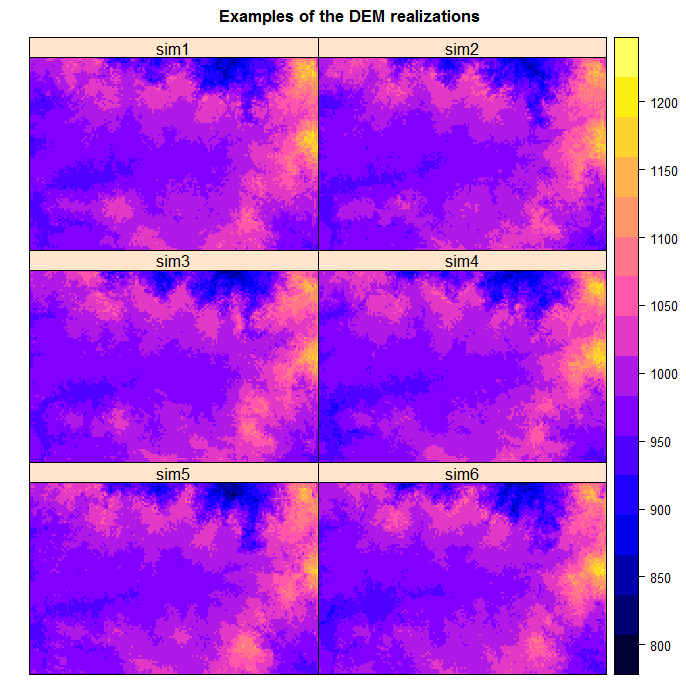We can view the mean and standard deviation of the sampled elevation. If the sample size was very large then the sample mean would be close to ‘dem30m’ and the sd close to ‘dem30m_sd’:

# compute and plot the slope sample statistics
# e.g. mean and standard deviation
dem_sample_mean <- mean_MC_sgdf(dem_sample)
dem_sample_sd <- sd_MC_sgdf(dem_sample)
grid.arrange(spplot(dem_sample_mean, main = list(label = "Mean of the DEM realizations", cex = 1)),
spplot(dem_sample_sd, main = list(label = "Standard dev. of the DEM realizations", cex = 1)))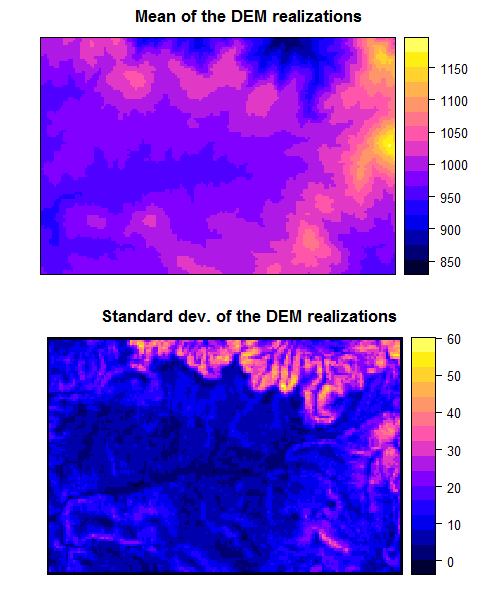The spatial pattern of the generated realizations depends on the degree of spatial autocorrelation. For instance, notice that the realizations become more ‘noisy’ if we assume less spatial autocorrelation:

dem_crm2 <- makeCRM(acf0 = 0.2, range = 300, model = "Exp")
demUM2 <- defineUM(uncertain = TRUE, distribution = "norm", distr_param = c(dem30m, dem30m_sd), crm = dem_crm2)
dem_sample2 <- genSample(UMobject = demUM2, n = 100, samplemethod = "ugs", nmax = 20, asList = FALSE,
debug.level = -1)
[using unconditional Gaussian simulation]

25% done
48% done
72% done
96% done
100% done
grid.arrange(spplot(dem_sample, c(1), main = list(label = "dem_sample, acf0 = 0.8, range = 300m", cex = 1)),
spplot(dem_sample2, c(1), main = list(label = "dem_sample2, acf0 = 0.2, range = 300m", cex = 1)))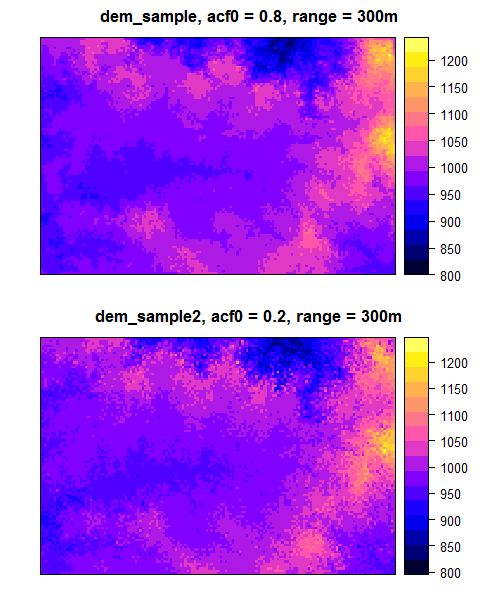Can you spot the difference? Higher auto-correlation yields ‘smoothened’ realizations. Lower values produce a more ‘noisy’ field.

#### Uncertainty propagation through the model that calculates slope using elevation as input

In order to perform uncertainty propagation analysis using ‘spup’, the model through which uncertainty is propagated needs to be defined as an R function. The ‘DEM’ data object includes an example of a pre-defined model that calculates slope using elevation as input. In this case the function is based on the terrain() function from the raster package.

# view the model
Slope
function (DEM, ...)
{
require(raster)
demraster <- DEM %>% raster()
demraster %>% terrain(opt = "slope", unit = "degrees", ...) %>%
as("SpatialGridDataFrame")
}

The propagation of uncertainty occurs when the model is run with an uncertain input. Running the model with a sample of realizations of uncertain input variable(s) yields an equally large sample of model outputs that can be further analysed. To run the Slope model with the elevation realizations we use the propagate() function. The propagate() function takes as arguments:

• a sample from the uncertain model inputs and any other remaining model inputs and parameters as a list.
• the model as a function in R.
• the number of Monte Carlo runs. This can be equal or smaller than the number of realizations of the uncertain input variable(s).

In order to run the propagation function it is necessary to save the sample of an uncertain input variable in a list. We can either coerce the existing ‘dem_sample’ object or get it automatically by setting up the ‘asList’ argument of genSample() to TRUE.

# coerce  SpatialGridDataFrame to a list of individual SpatialGridDataFrames
dem_sample <- map(1:ncol(dem_sample), function(x){dem_sample[x]})

# or sample from uncertain input and save it in a list
dem_sample <- genSample(UMobject = demUM, n = 100, samplemethod = "ugs", nmax = 20, asList = TRUE,
debug.level = -1)
[using unconditional Gaussian simulation]

26% done
51% done
76% done
100% done
# run uncertainty propagation
slope_sample <- propagate(dem_sample, model = Slope, n = 100)

#### Visualization of results

We can now view the sample of model output realizations (i.e. slope) and visualize uncertainty by calculating and plotting the sample mean and standard deviation. In our case we need to coerce the output of the propagationfunction saved as a list back to a SpatialGridDataFrame.

# coerce slopes list to a SpatialGridDataFrame
s <- slope_sample[]
for (i in 2:length(slope_sample)) {
s@data[i] <- slope_sample[[i]]@data
}
names(s@data) <- paste("slope", c(1:ncol(s)), sep = "")
slope_sample <- s
rm(s)

# view the sample of the model output
spplot(slope_sample[c(5,6,3,4,1,2)], main = list(label = "Examples of the slope realizations", cex = 1))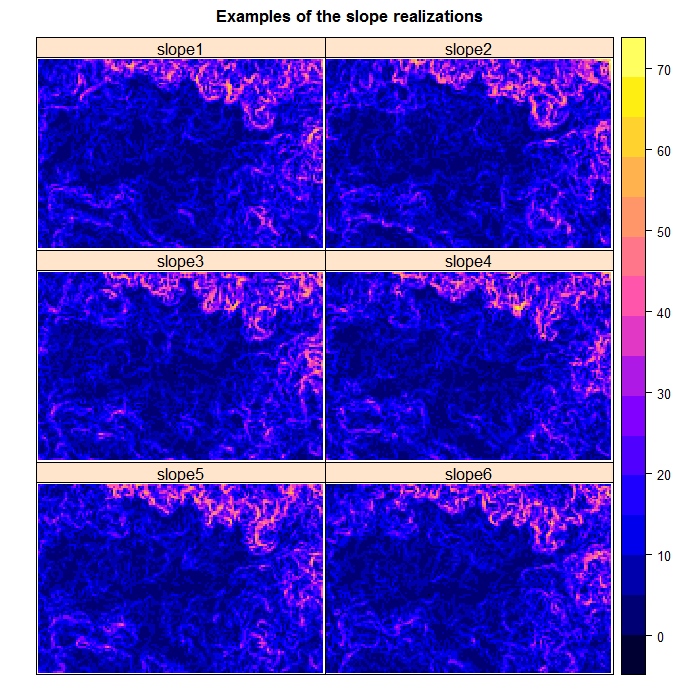# compute and plot slope sample statistics
# e.g. mean and standard deviation
slope_mean <- mean_MC_sgdf(slope_sample)
summary(slope_mean)
Object of class SpatialGridDataFrame
Coordinates:
min     max
s1 7394249 7398749
s2 4841999 4844999
Is projected: TRUE
proj4string :
[+init=epsg:3857 +proj=merc +a=6378137 +b=6378137 +lat_ts=0.0 +lon_0=0.0
+x_0=0.0 +y_0=0 +k=1.0 +units=m [email protected] +no_defs]
Grid attributes:
cellcentre.offset cellsize cells.dim
s1           7394264       30       150
s2           4842014       30       100
Data attributes:
mean_realizations
Min.   : 0.6908
1st Qu.: 6.2873
Median : 9.4023
Mean   :11.6496
3rd Qu.:14.9440
Max.   :41.8185
NA's   :496      
slope_sd <- sd_MC_sgdf(slope_sample, na.rm = TRUE) # na.rm = TRUE is necessary, because slope cannot be calculated at the border
grid.arrange(spplot(slope_mean, main = list(label = "Mean of the slope realizations [deg]", cex = 1)),
spplot(slope_sd, main = list(label = "Standard dev. of the slope realizations [deg]", cex = 1)))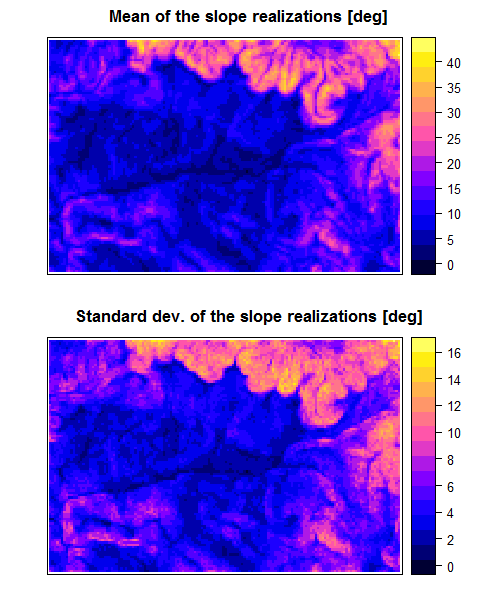We can view example of slope realizations at locations:

# select a couple of locations and plot points on the slope map
loc1 <- 2200  # the numbers correspond to rows number in SpatialGridDataFrame 'dem30m'
loc2 <- 6200  # with an example location of high and low DEM sd
points <- data.frame(t(coordinates(slope_sample)[loc1,]))
points[2,] <- t(coordinates(slope_sample)[loc2,])
coordinates(points) <- ~ s1 + s2
proj4string(points) <- proj4string(slope_sample)
spplot(slope_sample, c(1), col.regions = grey.colors(16),
sp.layout = c('sp.points', points, col = "red", pch = 19, cex = 1.2))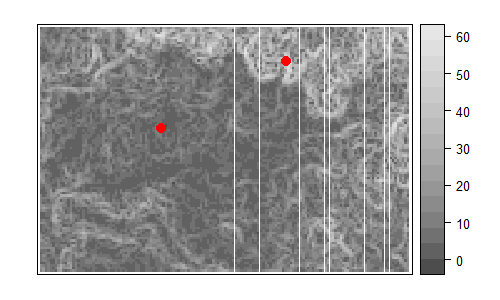l_mean <- mean(as.numeric(slope_sample@data[loc1,]))
l_sd <- sd(as.numeric(slope_sample@data[loc1,]))
h_mean <- mean(as.numeric(slope_sample@data[loc2,]))
h_sd <- sd(as.numeric(slope_sample@data[loc2,]))

par(mfrow = c(1,2))
hist(as.numeric(slope_sample@data[loc1,]), main = paste("Slope at high DEM sd,", "\n",
"mean = ", round(l_mean, 2), ", sd = ", round(l_sd, 2), sep = ""), xlab = "Slope")
hist(as.numeric(slope_sample@data[loc2,]), main = paste("Slope at low DEM sd,", "\n",
"mean = ", round(h_mean, 2), ", sd = ", round(h_sd, 2), sep = ""), xlab = "Slope")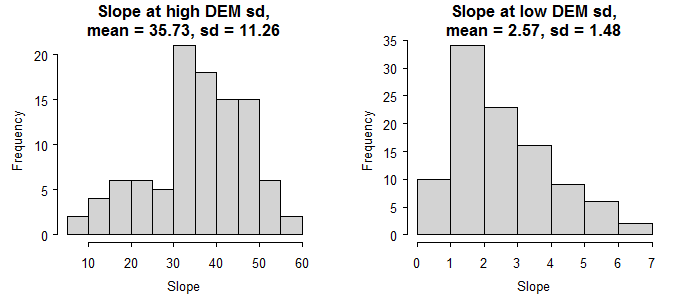Since slope is a nearly linear combination of elevation differences, the slope sd has a similar spatial pattern as elevation sd. Therefore at locations with high elevation sd we get high uncertainty in slope predictions.

# scatter plot of slope against elevation
slope1 <- Slope(dem30m)
names(slope1@grid@ cellcentre.offset) <- c("x", "y")
df <- cbind(dem30m_sd@data, slope1@data)
ggscatmat(data = df, alpha=0.15)
Warning: Removed 496 rows containing non-finite values (stat_density).
Warning: Removed 1 rows containing missing values (geom_text).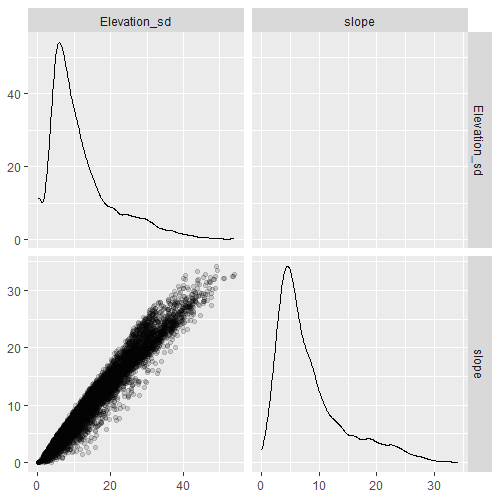We can also look at specific quantiles of the slope sample.

# or quantiles
slope_q <- quantile_MC_sgdf(slope_sample, probs = c(0.1, 0.25, 0.75, 0.9), na.rm = TRUE)
spplot(slope_q[c(3,4,1,2)], mail = list(label = "Quantiles of slope realizations", cex = 1))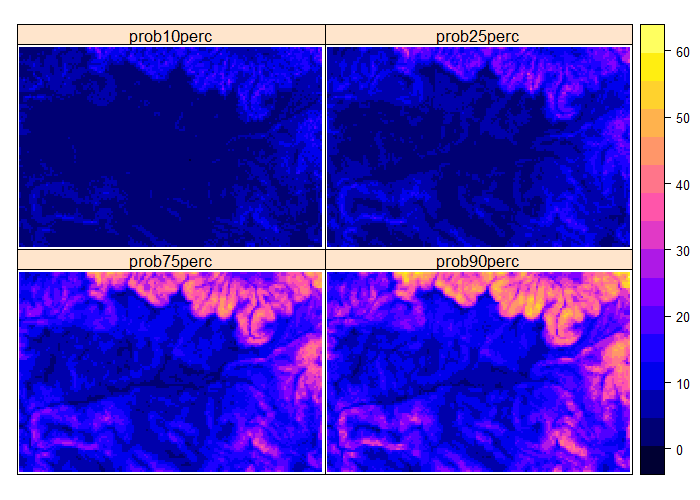For example, let us identify locations with slopes that we are 90% sure that are possible (slope > 5 deg.) for tourist skiing.

# identify areas of slope > 10 deg with 90% certainty.
slope_q$skiing <- NA slope_q$skiing <- ifelse(slope_q$prob10perc > 5, ">90%certain", "<90%certain") slope_q$skiing <- as.factor(slope_q$skiing) spplot(slope_q, "skiing", col.regions = c("red","green"), main = "Areas suitable for skiing")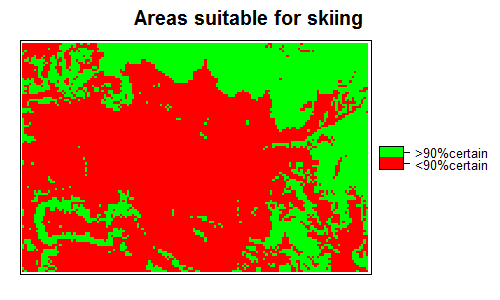Additionally to guarantee snow we can select areas with elevation > 1000m. # identify areas of elevation > 1000m and overlay with the slope map above dem30m$Elev1000 <- NA
dem30m$Elev1000[dem30m$Elevation > 1000] <- "snow"
dem30m$Elev1000[is.na(dem30m$Elev1000)] <- "no snow"
dem30m$Elev1000 <- as.factor(dem30m$Elev1000)

snow <- dem30m
snow_poly <- as(as(snow, "SpatialPixelsDataFrame"), "SpatialPolygonsDataFrame")
snow_poly <- aggregate(snow_poly, by = "Elev1000")

transparent_grey <- rgb(0,0,0, alpha = 0.35)
spl <- list("sp.grid", slope_q, col = c("red","green"))
spplot(snow_poly, col.regions = c(transparent_grey, "transparent"),
main = paste("Sufficiently certain that suitable", "\n", "for skiing and snow presence") ,
sp.layout = spl)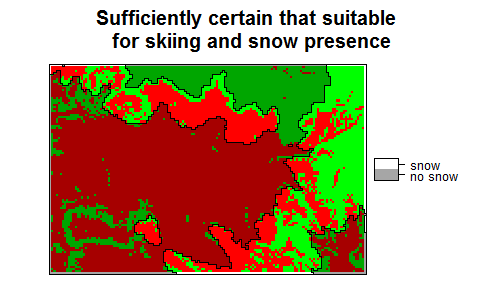### Acknowledgements

The Zlatibor dataset was kindly provided by Prof. Branislav Bajat from the University of Belgrade, Serbia.

This project has received funding from the European Union’s Seventh Framework Programme for research, technological development and demonstration under grant agreement no 607000.